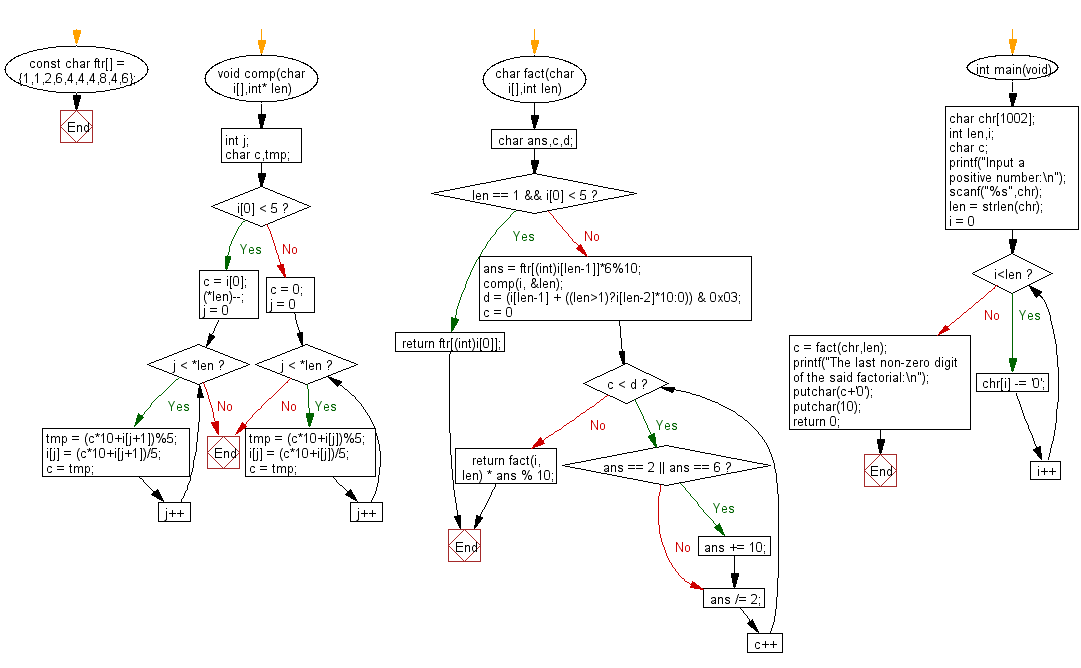﻿ C Program: Find the last non-zero digit of a factorial

# C Exercises: Find the last non-zero digit of the factorial of a given positive integer

## C Basic Declarations and Expressions: Exercise-92 with Solution

Write a C program to find the last non-zero digit of the factorial of a given positive integer.
For example for 5!, the output will be "2" because 5! = 120, and 2 is the last nonzero digit of 120

Sample Solution:

C Code:

``````#include <stdio.h>
#include <string.h>

// Array representing the factorials of numbers 0 to 9
const char ftr[] = {1,1,2,6,4,4,4,8,4,6};

// Function to perform computation on an array of digits
void comp(char i[], int* len)
{
int j;
char c, tmp;

if(i < 5)
{
c = i;
(*len)--;

// Iterate through the digits and perform computation
for(j = 0; j < *len; j++)
{
tmp = (c*10 + i[j+1]) % 5;
i[j] = (c*10 + i[j+1]) / 5;
c = tmp;
}
}
else
{
c = 0;

// Iterate through the digits and perform computation
for(j = 0; j < *len; j++)
{
tmp = (c*10 + i[j]) % 5;
i[j] = (c*10 + i[j]) / 5;
c = tmp;
}
}
}

// Recursive function to calculate factorial
char fact(char i[], int len)
{
char ans, c, d;

if(len == 1 &&i < 5)
return ftr[(int)i];
else
{
ans = ftr[(int)i[len-1]] * 6 % 10;
comp(i, &len);
d = (i[len-1] + ((len> 1) ? i[len-2] * 10 : 0)) & 0x03;

// Apply further calculations
for(c = 0; c < d; c++)
{
if(ans == 2 || ans == 6)
ans += 10;
ans /= 2;
}

return fact(i, len) * ans % 10;
}
}

int main()
{
char chr;
int len, i;
char c;

printf("Input a positive number:\n");
scanf("%s", chr);
len = strlen(chr);

// Convert character digits to actual integers
for(i = 0; i<len; i++)
chr[i] -= '0';

// Calculate factorial and get the last non-zero digit
c = fact(chr, len);

printf("The last non-zero digit of the said factorial:\n");
putchar(c + '0');

putchar(10);
return 0;
}
``````

Sample Output:

```Input a positive number:
The last non-zero digit of the said factorial:
0
```

Flowchart:C programming Code Editor:

What is the difficulty level of this exercise?

Test your Programming skills with w3resource's quiz.

﻿